# Word Problems with Multiplication and Division

##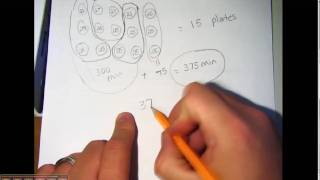By Mathademics

Word Problems with Multiplication and Division# Multiplication word problem example 1 | Multiplication and division | Arithmetic | Khan Academy

##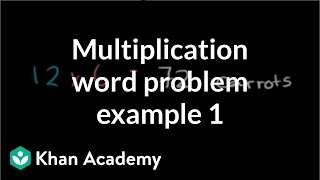By Khan Academy

Word problems on multiplication# Video for Lesson 16:Word Problems Involving Multiplication

##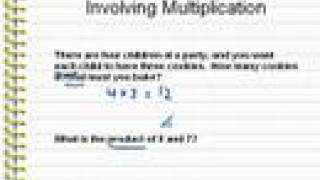By mathwithlarry

Learn about word problems using multiplication. The instruction is clear and encourages students to not be discouraged by word problems.# Video for Lesson 16: Word Problems Involving Multiplication

##By mathwithlarry

Learn about word problems using multiplication. The instruction is clear and encourages students to not be discouraged by word problems.# Multiplying with Multiplicative Comparison Word Problems

##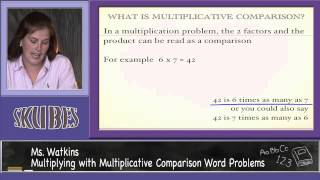By Skubes ed

Multiply or divide to solve word problems involving multiplicative comparison# Multiplication, division word problems: how many field goals? | Arithmetic | Khan Academy

##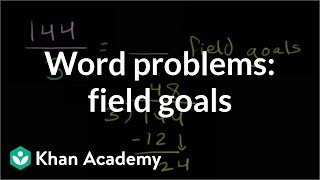By Khan Academy

It's time to start putting to practice the multiplication and division skills you've been building. Word problem fun!# Multiplication Multi-Step Word Problems

##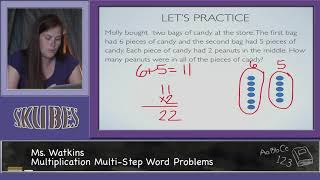By Skubes ed

Solve multistep word problems posed with whole numbers and having whole-number answers using the four operations# Division word problem example 1 | Multiplication and division | Arithmetic | Khan Academy

##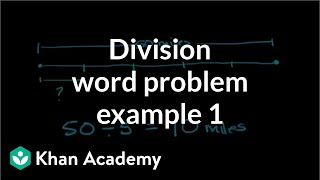By Khan Academy

Division of world problems# Multiplying Whole Numbers Framing Video

##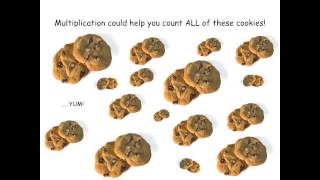By eSparkLearningVideos

This video narrates a word problem involving multiplication.# Multiplication, division word problems: how many pieces of pizzas? | Arithmetic | Khan Academy

##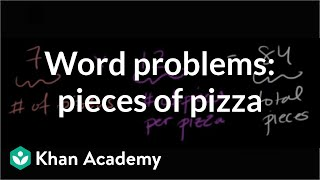By Khan Academy

How many pizzas, pieces per pizza, and eaten pieces were there? Oh, the questions we must answer! It's enough to make you hungry.# Comparing with multiplication word problems

##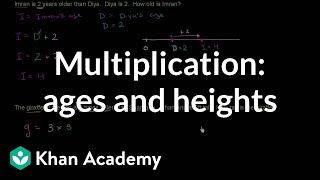By Khan Academy

You'll learn that sometimes multiplication is used as a way of comparing two things. In these 2 examples, we're comparing age and height.# Multi-step word problem with addition, subtraction, and multiplication | Pre-Algebra | Khan Academy

##By Khan Academy

world problems multiplication# Solving 2-Step Word Problems | Good To Know | WSKG

##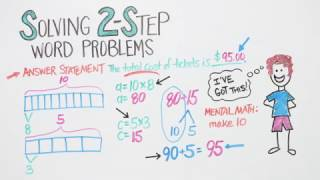By WSKG Public Media

solve two-step word problems using addition, subtraction, multiplication, and division.# [4.NF.4c-1.0] Word Problems: Multiplying - Common Core Standard

##By Freckle education

Solve word problems involving multiplication of a fraction by a whole number, e.g., by using visual fractions models and equations to represent the problem.# [4.NBT.5-1.0] Four digit multiplication - Common Core Standard - Word Problem

##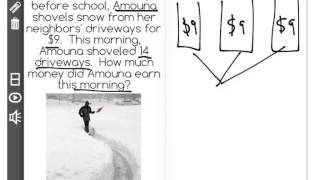By Freckle Education

Discover more Common Core Math at https://www.frontrowed.comMultiply a whole number of up to four digits by a one-digit whole number using strategies based on place value and the properties of operations. Illustrate and explain the calculation by using equations, rectangular arrays, and/or area models.Front Row is a free, adaptive, Common Core aligned math program for teachers and students in kindergarten through eighth grade. Front Row allows students to practice math at their own pace - learning advanced concepts when they 're ready and receiving remediation when they struggle. Front Row provides teachers with access to a detailed data dashboard and weekly email reports that show which standards are causing students difficulty, what small groups can be formed for interventions, and how their students are progressing in math.Discover more Common Core Math at https://www.frontrowed.com##By Lumos Learning

Grade 4 lesson demonstrating CCSS Math Standard 4.OA..A.1,2, using literacy strategies to understand word problems. Lesson progresses through prior understandings of multiplication as groups of objects to multiplicative comparisons.##By

Grade 4 lesson demonstrating CCSS Math Standard 4.OA..A.1,2, using literacy strategies to understand word problems. Lesson progresses through prior understandings of multiplication as groups of objects to multiplicative comparisons.# Multi-step rational number word problems

##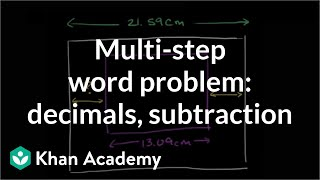By Khan Academy

First visualize this word problem then use subtraction and multiplication of decimals and fractions to get at the answer.# Multi-step word problem with decimals and subtraction

##By Khan Academy

First visualize this word problem then use subtraction and multiplication of decimals and fractions to get at the answer.# Multi-step word problem with decimals and subtraction

##By Khan,Academy

First visualize this word problem then use subtraction and multiplication of decimals and fractions to get at the answer.